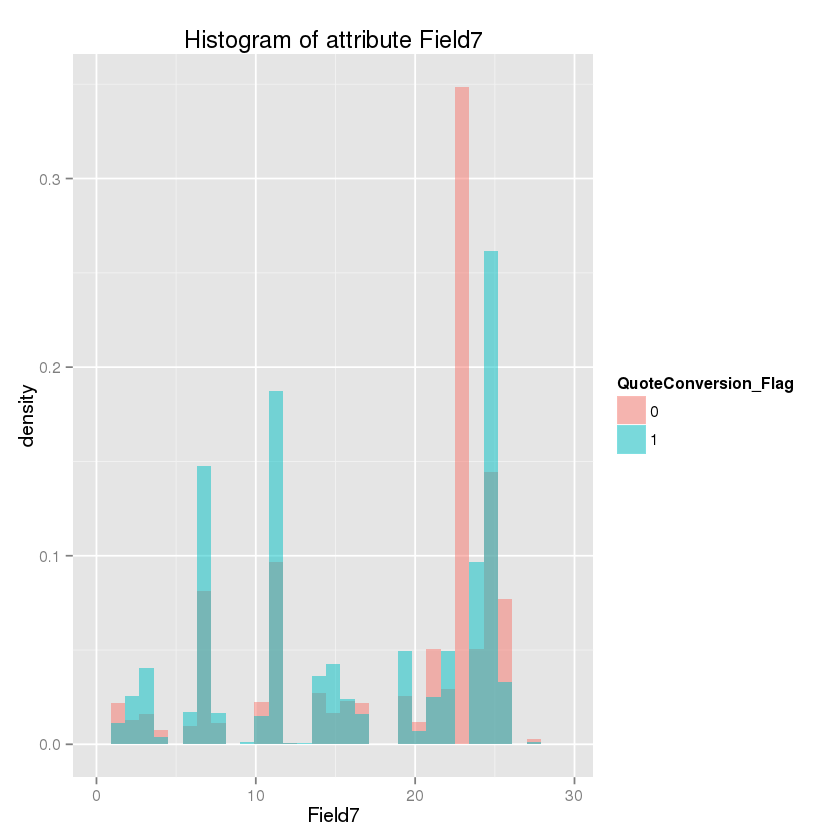### Long H. Nguyen

Knowledge worths sharing!

Email

# R snippet code

My collection of small and re-usable source code for R.

Feel free to use it if you find it helpful.

# Correlation plot

It could be the first step of your data analytics project. You need to visually pair-wise distribution of your data and how the variables are correlated to each others.

``````corrgram(data,
lower.panel=panel.pts, upper.panel=panel.cor,
diag.panel=panel.density)
``````

# Overlay data distributions for comparisons

Given two groups of data, we may need to compare their distributions. Overlay distribution plots will be helpful with beautiful visualization.

``````# assign two groups for legends
group1\$partition = 'group1'
group2\$partition = 'group2'
pltData = rbind(group1,group2)
group1\$partition = NULL
group2\$partition = NULL
for( var in imp_vars){
xl = paste(var,'\n')
xl = paste(xl, 'group1: ',round(mean(group1[[var]],na.rm = T),2), '+/-', round(sd(group1[[var]],na.rm = T),2),'\n')
xl = paste(xl, 'group2: ',round(mean(group2[[var]],na.rm = T),2), '+/-', round(sd(group2[[var]],na.rm = T),2),'\n')
print(ggplot(pltData, aes(pltData[[var]], fill = partition)) + geom_density(alpha = 0.2) + xlab(xl))
cat(xl)
}
``````

# Histogram of important features vs label

One of my sample code taken from a Kaggle competition.

It is very helpful to create ‘golden’ features. :)

``````library(readr)
library(ggplot2)
library(ggthemes)
#This important list is generated by my model, here I just provide top 10.
important_list = c('PropertyField37','SalesField5','PersonalField9','Field7'
,'PersonalField2','PersonalField1','SalesField4','PersonalField10A'
, 'SalesField1B', 'PersonalField10B', 'PersonalField12')
train\$QuoteConversion_Flag = as.factor(train\$QuoteConversion_Flag)
for( att in important_list){
#Density histogram
plot <- ggplot(train, aes_string(att, fill = 'QuoteConversion_Flag')) +
geom_histogram(alpha = 0.5, position = 'identity') +
ggtitle(paste0('Histogram of attribute ', att))
}
``````

For example, Field7 at bin value ~25 could be useful for creating an additional variable.